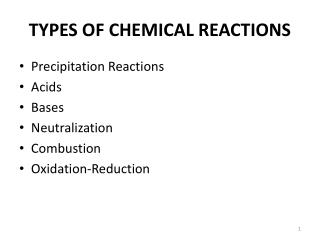DownloadDownload PresentationTYPES OF CHEMICAL REACTIONS

# TYPES OF CHEMICAL REACTIONS

Télécharger la présentation## TYPES OF CHEMICAL REACTIONS

- - - - - - - - - - - - - - - - - - - - - - - - - - - E N D - - - - - - - - - - - - - - - - - - - - - - - - - - -
##### Presentation Transcript

1. TYPES OF CHEMICAL REACTIONS • Precipitation Reactions • Acids • Bases • Neutralization • Combustion • Oxidation-Reduction

2. 2Mg 2Mg2+ + 4e- O2 + 4e- 2O2- 2Mg + O2 + 4e- 2Mg2+ + 2O2- + 4e- 2Mg + O2 2MgO Oxidation-Reduction Reactions (electron transfer reactions) Oxidation half-reaction (lose e-) Reduction half-reaction (gain e-)

3. Types of Chemical ReactionsOxidation Reduction Reactions • Electrons are transferred • Oxidation: Loss of Electrons • Reduction: Acceptance of Electrons

4. Cu (s) + 2AgNO3 (aq)  Cu(NO3)2 (aq) + 2Ag (s) Zn (s) + CuSO4 (aq)  ZnSO4 (aq) + Cu (s)

5. Types of Re Dox ReactionsSingle Displacement With Metals • Zn + HCl  ZnCl2 + H2 • A + BC  AC + B • Li + HOH  • Ca + HNO3 

6. A + BC AC + B Sr + 2H2O Sr(OH)2 + H2 TiCl4 + 2Mg Ti + 2MgCl2 Cl2 + 2KBr 2KCl + Br2 Types of Oxidation-Reduction Reactions Displacement Reaction +1 +2 0 0 Hydrogen Displacement +4 0 0 +2 Metal Displacement -1 0 0 -1 Halogen Displacement

7. A + B C 2Al + 3Br2 2AlBr3 C A + B 2KClO3 2KCl + 3O2 Types of Oxidation-Reduction Reactions Combination Reaction +3 -1 0 0 Decomposition Reaction +1 +5 -2 +1 -1 0

8. A + O2 B S + O2 SO2 2Mg + O2 2MgO Types of Oxidation-Reduction Reactions Combustion Reaction +4 -2 0 0 +2 -2 0 0

9. M + BC MC + B Ca + 2H2O Ca(OH)2 + H2 Pb + 2H2O Pb(OH)2 + H2 The Activity Series for Metals Hydrogen Displacement Reaction M is metal BC is acid or H2O B is H2

10. Problem 4.54 • PREDICT THE OUTCOME (COMPLETE THE PRODUCT SIDE) FOR THE FOLLOWING REACTIONS AND BALANCE THE EQUATIONS • (a) Cu (s) + HCl (aq)  • (b) I2(s) + NaBr (aq)  • (c ) Mg (s) + CuSO4(aq)  • (d) Cl2(g) + KBr4(aq) 

11. Oxidation number The charge the atom would have in a molecule (or an ionic compound) if electrons were completely transferred. • Free elements (uncombined state) have an oxidation number of zero. Na, Be, K, Pb, H2, O2, P4 = 0 • In monatomic ions, the oxidation number is equal to the charge on the ion. Li+, Li = +1; Fe3+, Fe = +3; O2-, O = -2 • The oxidation number of oxygen isusually–2. In H2O2 and O22- it is –1. 4.4

12. The oxidation number of hydrogen is +1except when it is bonded to metals in binary compounds. In these cases, its oxidation number is –1. • Group IA metals are +1, IIA metals are +2 and fluorine is always –1. 6. The sum of the oxidation numbers of all the atoms in a molecule or ion is equal to the charge on the molecule or ion. 7. Oxidation numbers do not have to be integers. Oxidation number of oxygen in the superoxide ion, O2-, is –½. HCO3- O = –2 H = +1 What are the oxidation numbers of all the elements in HCO3- ? 3x(–2) + 1 + ? = –1 C = +4

13. The Oxidation Numbers of Elements in their Compounds